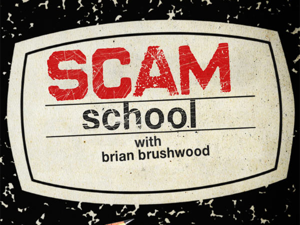0

## Math vs. Sorcery

Published on Thursday, January 24, 2013 in , , , , ,Imagine you and a friend are performing some of the feats you've learned from, say, here on Grey Matters, on Scam School or from James Grime, and you're accused of witchcraft! You're hauled off by the authorities, and given a test to see if there's a supernatural connection between the two of you.

You and your friend are put in separate rooms, and each of you is given a single playing card taken from a shuffled deck. You must state whether you believe that your friend has been dealt a card of the same color or a different color. Naturally, your friend is asked the same question.

The judgement of the outcome, however, is very harsh. If both you and your friend make correct statements, that is considered sufficient proof of supernatural powers, and you are put to death. If both you and your friend make incorrect statements, you are released and the charges of witchcraft are dropped. If one makes a correct statement and the other makes an incorrect statement, the results are considered inconclusive, and the two of you are tested again, up to 26 times (since a pair of cards are used each time, and there's 26 pairs of cards in a standard deck).

Considering that, at any point when your guesses match, you would be put to death, it's probably a good idea at this point to consider probabilities of survival.

If you and your friend just make random guesses as to the other's card, what are the chances of surviving?

Technically, if the cards aren't returned to the deck after each round of guessing, you'd have to do some math to work out the probabilities of the distribution of cards remaining in the deck at each point, but we're not going to take that consideration. In our calculations, we'll assume that the cards from each round are returned to the deck, and the deck is shuffled again. In mathematical terms, the dealing of 2 cards in each round will be considered an independent event, as opposed to a dependent event.

If you and your friend make a random guess on the first round, there's a 25% chance of you both being correct, and therefore being killed. There's a 25% chance of you being set free, and a 50% chance of being required to take another test.

Note that this means you have a 75% chance of surviving the first round, but only a 50% chance of being required to being submitted to another round of testing.

That's simple enough, but how do we take the 2nd round into account? For the second round, you still have a 25% chance of dying, but you only have a 50% of getting to that test in the first place. You add the 25% of dying in the first round to the 50% × 25% chance of dying in the second round. We work this out as .25 + (.50 × .25) = .25 + .125 = .375, or a 37.5% chance of being killed by the end of a second round.

For a third round, the logic is the same, and the equation becomes .25 + (.50 × .25) + (.502 × .25) = .25 + .125 + (.25 × .25) = .25 + .125 + .0625 = .4375, or a 43.75% chance of dying after 3 rounds.

Notice that each time, the chance of dying is increasing, so already this test doesn't look promising. Also note that the 50% chance for any given step is effectively the number of the round minus 1. This is even true for round 1, since 120 = 1 (Why is that, anyway?).

So, for 26 rounds, we have .25 + (.50 × .25) + (.502 × .25) + ... + (.5024 × .25) + (.5025 × .25). The mathematical shorthand for this is:

Running this through Wolfram|Alpha, we find that the probability of being put to death by the 26th round is 0.499999992549419403076171875, or a roughly 49.99% chance. That's not much better than a “heads you live, tails you die” coin flip.

Is it possible to improve on the 50/50 odds of survival? Fortunately, yes it is, and Scam School's Brian Brushwood, with the help of a friend, prove they can survive through all 26 rounds. Can you guess how they do it before their secret is revealed?

With all the complex math, it seems like the answer should be equally complex, but the answer is dead simple. When you think about it, they approach used in the video above must ALWAYS render the test inconclusive, thus allowing them to live through 26 tests.

You might notice that, contrary to the initial description of the problem, the cards aren't returned to the deck before the next round. However, the strategy nullifies the chances of dying in the first place, so the importance of the changing deck composition isn't important any longer.